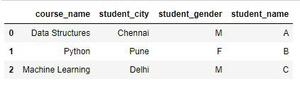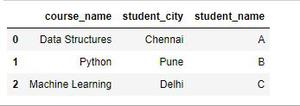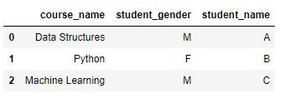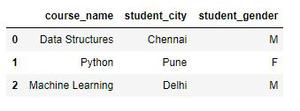# Select all columns, except one given column in a Pandas DataFrame

• Last Updated : 20 Aug, 2020

DataFrame Data structure are the heart of Pandas library. Dataframes are basically two dimension Series object. They have rows and columns with rows representing the index and columns representing the content. Now, let’s see how to Select all columns, except one given column in Pandas Dataframe.

First, Let’s create a Dataframe:

Attention geek! Strengthen your foundations with the Python Programming Foundation Course and learn the basics.

To begin with, your interview preparations Enhance your Data Structures concepts with the Python DS Course. And to begin with your Machine Learning Journey, join the Machine Learning - Basic Level Course

## Python3

 `# import pandas library``import` `pandas as pd`` ` `# create a Dataframe``data ``=` `pd.DataFrame({``    ``'course_name'``: [``'Data Structures'``, ``'Python'``,``                    ``'Machine Learning'``],``    ``'student_name'``: [``'A'``, ``'B'``, ``                     ``'C'``],``    ``'student_city'``: [``'Chennai'``, ``'Pune'``, ``                     ``'Delhi'``],``    ``'student_gender'``: [``'M'``, ``'F'``,``                       ``'M'``] })``# show the Dataframe``data`

Output:DataFrame

Method 1: Using Dataframe.loc[ ].

This GeeksForGeeks Dataframe is just a two dimension array with numerical index. Therefore, to except only one column we could use the columns methods to get all columns and use a not operator to exclude the columns which are not needed. This method works only when the Dataframe is not multi indexed (did not have more than one index).

Example: Select all columns, except one ‘student_gender’ column in Pandas Dataframe.

## Python3

 `# import pandas library``import` `pandas as pd`` ` `# create a Dataframe``data ``=` `pd.DataFrame({``    ``'course_name'``: [``'Data Structures'``, ``'Python'``,``                    ``'Machine Learning'``],``    ``'student_name'``: [``'A'``, ``'B'``, ``                     ``'C'``],``    ``'student_city'``: [``'Chennai'``, ``'Pune'``, ``                     ``'Delhi'``],``    ``'student_gender'``: [``'M'``, ``'F'``,``                       ``'M'``] })``                        ` `df ``=` `data.loc[ : , data.columns !``=` `'student_gender'``]`` ` `# show the dataframe``df`

Output:filtered student_gender column

Method 2: Using drop() method.

Dataframe supports drop() method to drop a particular column. It accepts two arguments, column/row name and axis

Example: Select all columns, except one ‘student_city’ column in  Pandas Dataframe.

## Python3

 `# import pandas library`` ` `import` `pandas as pd`` ` `# create a Dataframe``data ``=` `pd.DataFrame({``   ``'course_name'``: [``'Data Structures'``, ``'Python'``,``                   ``'Machine Learning'``],`` ` `   ``'student_name'``: [``'A'``, ``'B'``,``                    ``'C'``],`` ` `   ``'student_city'``: [``'Chennai'``, ``'Pune'``,``                    ``'Delhi'``],`` ` `   ``'student_gender'``: [``'M'``, ``'F'``,``                      ``'M'``] })`` ` `# drop method``df ``=` `data.drop(``'student_city'``,``               ``axis ``=` `1``)``  ` `# show the dataframe``df`

Output:student_city column removed

Method 3: Using Series.difference() method and [ ] operator together.

Series.difference() Method returns a new Index with elements from the index that are not in other.

Example: Select all columns, except one ‘student_name’ column in Pandas Dataframe.

## Python3

 `# import pandas library``import` `pandas as pd`` ` `# create a Dataframe``data ``=` `pd.DataFrame({``    ``'course_name'``: [``'Data Structures'``, ``'Python'``,``                    ``'Machine Learning'``],``    ``'student_name'``: [``'A'``, ``'B'``, ``                     ``'C'``],``    ``'student_city'``: [``'Chennai'``, ``'Pune'``, ``                     ``'Delhi'``],``    ``'student_gender'``: [``'M'``, ``'F'``,``                       ``'M'``] })``                        ` `df ``=` `data[data.columns.difference([``'student_name'``])]`` ` `# show the dataframe``df`

Output:filtered student_name column

My Personal Notes arrow_drop_up